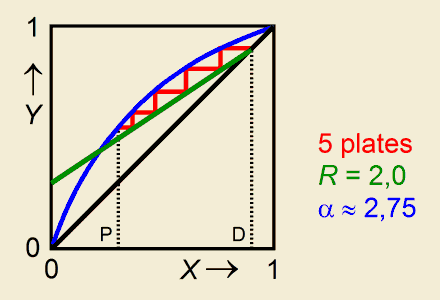# Batch RectificationCalculation of the Distillate Compositiona program for the Texas Instruments TI-83and TI-84 (Plus) graphing calculators

### Distillation in a column with a given number of theoretical plates

The boiling liquid is a mixture of two volatile components, wherein the mole fraction of the more volatile component is symbolized by X (hence 1–X matches the less volatile component).
Given values for:
• the relative volatility α,
• the composition XP of the boiling mixture in the pot,
• the number of theoretical plates in the distillation column inclusive of the pot,
• and the reflux ratio R,
the program calculates the composition XD of the drawn off distillate as well as all other liquid and vapour compositions X and Y belonging to the theoretical plates (Y is the mole fraction of the more volatile component in a vapour phase).
The calculations are according to the McCabe-Thiele method.### Program Listing

Elucidation and Help
PROGRAM:RECTIBD Title
ClrHome > PRGM > I/O > ClrHome
Disp "REL.VOLATILITY" (α>1)
Input A
max(A,1)→A > MATH > NUM > max(
"→" Symbolizes the STO> key
Disp "X POT MIXTURE" Composition of the boiling mixture. X is the mole fraction of the more volatile component of the liquid (0<X<1).
Input M
min(M,1)→M
Disp "NUMBER OF PLATES" Number of theoretical plates inclusive of the pot
Input P
Disp "REFLUX RATIO"
Input R
{P,3}→dim([A]) > 2nd, MATRX > MATH > dim(
> 2nd, MATRX > NAMES > [A]
1→B:M→C
ClrHome
Lbl 1 > PRGM > CTL > Lbl
Beginning of iteration loop
If B–C<.00001
Goto 2
(B+C)/2→D:D→X
For(N,1,P)
(RX+D)/(R+1)→Y Y is the mole fraction of the more volatile component of the vapour (0<Y<1).
Y/(Y+A(1–Y))→X Y and X are at equilibrium
N→[A](N,1)
X→[A](N,2)
Y→[A](N,3)
End
If X<M
Then
D→C
Else
D→B
End
Goto 1
Lbl 2
round((B+C)/2,2)→D
Disp "X DISTILLATE",D,"","PLATE, X, Y  >>" Distillate composition
Pause
round([A],2)→[A]
Disp [A] Compositions (first seven plates)
Pause :ClrHome
Disp "X,Y IN MAT [A]" Merely a reminder

After running and quitting the program, you still can have access to the liquid and vapour compositions X and Y of all plates. This may especially be of intrest when the number of plates exceeds seven. You'll find them stored in matrix [A] (> 2nd, MATRX > NAMES > [A], ENTER, ENTER).

### Example

Consider a mixture of benzene and toluene.

Input values:
• relative volatility = 2,55
XP (boiling mixture in the pot) = 0,60
• number of theoretical plates = 4 (that is 3 plates in the column)
• reflux ratio = 4

Output:
XD (distillate) = 0,97
Plate    X         Y
1       0,93     0,97       (top of the column)
2       0,86     0,94
3       0,75     0,88
4       0,60     0,79       (pot)

The vapour with composition Y3 = 0,88 is ascending from plate 3.
X3 = 0,75 is the composition of the liquid on plate 3. Both phases are at equilibrium.

(To retrieve the plate compositions as shown in an example coming with the program RECTIBP, put in XP = 0,58.)

Hein ten Horn
Top | Programs Texas Instruments | Home